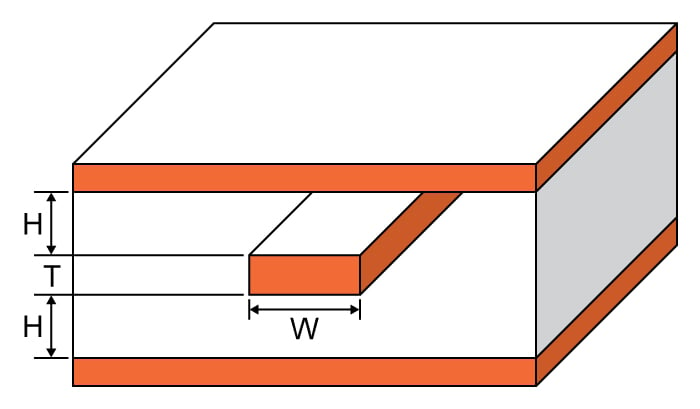# Stripline Trace Width Calculator

## A calculator designed to calculate the trace width of a stripline based on the maximum current rating

Amps
°C
Ohms
Volts
Watts

### Overview

The maximum current that a stripline can safely handle is a function of its width. For this reason, trace width is important in the design of striplines. This calculator computes the trace width of a stripline if the maximum current, trace thickness, temperature rise, ambient temperature and length are given. This tool will also compute the resistance of the stripline, the voltage drop on it, and the resulting power dissipation.### Equations

$$I_{max} = \sqrt{ \frac{\Delta T}{215.3W^{-1.15} T^{-1.0}}}$$

$$R = \frac{\rho_{cu} L}{A} (1+\alpha_{cu} \Delta T)$$

$$V = I_{max} R$$

$$P = V I_{max}$$

Where:

$$\Delta T$$ = temperature rise

$$W$$ = trace width

$$T$$ = trace thickness

$$L$$ = trace length

$$\rho_{cu}$$ = resistivity of Copper = 1.7 x 10-6

$$\alpha_{cu}$$ = temperature coefficient of Copper = 3.9 x 10-3

*The maximum current formula is derived through curve fitting data found on IPC-2221

### Applications

Striplines are modified PCB traces where the conductor is sandwiched between two conducting planes and whose dimensions are carefully calculated to achieve a desired circuit operation. These devices arose because of the need for a much more efficient impedance device to be used in very high frequencies.  They are efficient because they don't radiate signal, as the conductor is well protected. They are convenient because striplines are easier to manufacture compared to standard capacitors and inductors.

Just like the standard capacitor and inductor, the stripline is also subject to maximum ratings. This tool uses the relationship between the ampacity of a conductor and its dimensions. A wider trace means a lower resistance, which also means a higher current. A conductor has its limits when it comes to the current it can handle, limiting how wide the trace of a stripline can be.